Thermodynamics and Propulsion

# 7.5 Numerical Example of the Approach to the Equilibrium Distribution

Reynolds and Perkins give a numerical example which illustrates the above concepts and also the tendency of a closed isolated system to tend to equilibrium. The starting point is a system in an initial microscopic state that is not an equilibrium distribution. We expect the system will change quantum state, with disorder, randomness growing until they reach the equilibrium values. The specific system to be studied is composed of 10 particles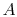,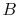,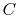, ...,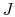, each of which can exist in one of 5 states, of energies 0, 1, 2, 3, 4. The system is isolated and has a total energy of 30. The total energy remains unchanged during the evolution of the microscopic states. Some of the allowed states are shown in Figure 7.1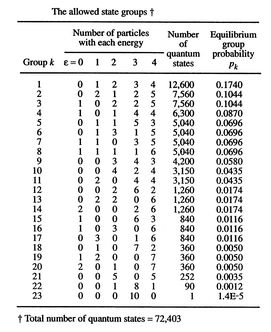For ten particles, 4 energy states, and a total energy of 30, there are 72,403 possible quantum states (4 states are indicated in Figure 7.1). However, there are only 23 possible distributions in terms of the number of particles having a given energy as shown in Figure 7.2. For example, states 2 and 3 in Figure 7.1 are two different quantum states, but they represent the same group (22) in Figure 7.2. The allowed state groups

If the quantum-state probabilities are equal, each quantum state has a probability of 1/72,403. The probabilities of each group are thus directly proportional to the number of quantum states in this group. For instance, group 22 has 90 quantum states, so its probability is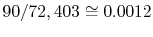. We now know what the equilibrium distribution of probabilities is. We now address the time evolution of a system to the equilibrium state. To see this, we start a system from one of the 22 non-equilibrium groups and track the behavior over time. A way to examine the process is to consider what happens if two particles interact, doing this numerically for the instantaneous quantum state. The two particles are free to change energy as long as the total energy of the system is conserved. This may or may not end up by changing the state group (the particles could interact and only switch states). There are 45 possible pairs for this interaction (there are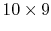possible ways to carry out the interaction, but two of them, say interactions betweenand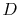andand, are the same), and we assume that any of them is equally likely to happen.

If the system is initially in state 1 of Figure 7.1, it is in group 23 of Figure 7.2. For each of the 45 pairs, there are two interactions that take the system to group 22, and one that leaves the system unchanged. (For interactions betweenand, say, the result can be thatandhave their energy unchanged, thatloses energy andgains energy, or thatgains energy andloses energy. In the first of these, the system will remain in group 23. In the second and third it will move to group 22.) Hence the transition probability from group 23 to group 22 is, and the transition probability from 23 to 23 is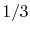.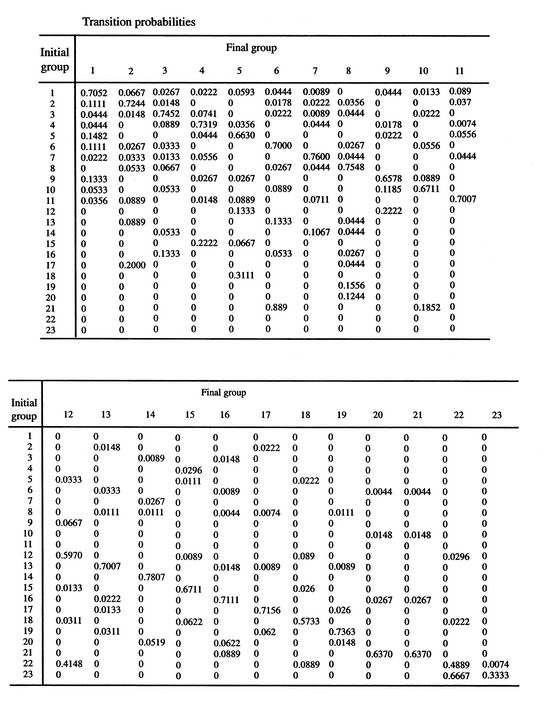For the other groups, the transitions are more complicated, but can be found numerically, with the results shown in Figure 7.3. The numerical experiments were carried out with the system initially in state 23 and with successive interactions chosen randomly in accordance with the transition probabilities of Figure 7.3. The experiment was repeated 10,000 times, with a different group history traced out each time and, again, the system energy maintained at 30. The fraction of the experiments in which each group occurred at time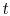was used to calculate the group probabilities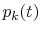at each time. The entropy was then found for the distribution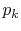at that time.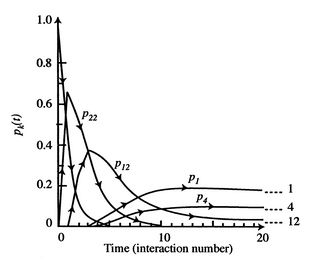Figure 7.4 shows the evolution of some of thewith time (the unit of time is the interaction number for the calculations) starting from group 23. After roughly ten interactions, the probabilities have reached a steady-state level, which are the equilibrium probabilities from Figure 7.2.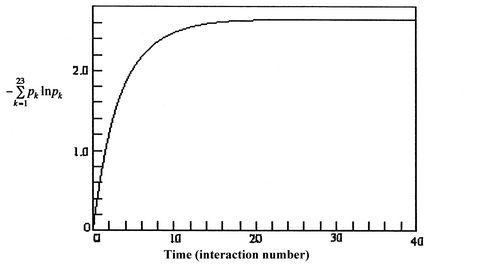The computed entropy is given in Figure 7.5 as a function of time. It increases to the equilibrium value with the same sort of behavior as the probability distribution.

The interactions allow the system to change groups. The transition probabilities are large for groups with high equilibrium probabilities.

There is one additional aspect of the behavior that is brought out in the text. This is the difference in overall probabilities between the order of transitions. The probability of a transition sequence is the product of the individual step transition probabilities. The transition 23-22-12-9-1 thus has the probability: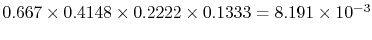. The reverse transition, 1-9-12-22-23 has the probability: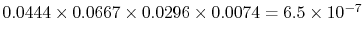. There is an enormous probability that the system will move towards (and persist in) quantum state groups that have high equilibrium probabilities. Once a system has moved out of group 23, there is little likelihood that it will ever return. Further, for engineering systems, which have not 10 particles, but upwards of, the difference between transitions and their reverses are much more marked, and the probability is overwhelming that the distribution will be a quantum state with a broad distribution of particle energies.

UnifiedTP